# Ampère's Law

(redirected from Ampere's law)

## Ampère's law

A law of electromagnetism which expresses the contribution of a current element of length dl to the magnetic induction (flux density) B at a point near the current. Ampère's law, sometimes called Laplace's law, was derived by A. M. Ampère after a series of experiments during 1820–1825.

Whenever an electric charge is in motion, there is a magnetic field associated with that motion. The flow of charges through a conductor sets up a magnetic field in the surrounding region. Any current may be considered to be broken up into infinitesimal elements of length dl, and each such element contributes to the magnetic induction at every point in the neighborhood. The contribution dB of the element is found to depend upon the current I, the length dl of the element, the distance r of the point P from the current element, and the angle Θ between the current element and the line joining the element of the point P (see illustration). Ampère's law expresses the manner of the dependence by Eq. (1). The field near

(1)a current may be calculated by finding the vector sum of the contributions of all the various elements that make up the current.

The proportionality factor k depends upon the units used in Eq. (1) and upon the properties of the medium surrounding the current. In the SI system, the factor k is assigned a value of 10-7 weber/ampere-meters when the current is in empty space. As in other equations associated with electric and magnetic fields, for example Coulomb's law, it is convenient to replace k by a new factor μ0 related to k as in Eq. (2).

(2)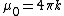This substitution removes the factor 4π from many derived equations in which it would otherwise appear. With this substitution Ampère's law becomes Eq. (3). The factor μ0 is called the
(3)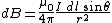permeability of empty space.

The direction of dB at each point may be described in terms of a right-hand rule. If the current element is grasped by the right hand with the thumb pointing in the direction of the current, the fingers encircle the current in the direction of the magnetic induction.

McGraw-Hill Concise Encyclopedia of Physics. © 2002 by The McGraw-Hill Companies, Inc.
The following article is from The Great Soviet Encyclopedia (1979). It might be outdated or ideologically biased.

## Ampère’s Law

the law of mechanical (ponderomotive) interaction between two currents flowing in short segments of conductors which are at some distance from each other.

The force F12 acting from first conductor segment Δ/1 on the second Δ/2 (see Figure 1) is equal to: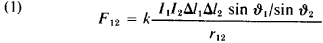The distance between the segments r12 is regarded as the path from the first segment to the second, and the directions of the segments refers to the directions of the currents I1 and I2 flowing in them; &1 is the angle between the directions of Δ/1 and r12; ϑ2 is the angle between Δ/2 and the perpendicular η to the plane containing Δl1 and r12 (the direction of η coincides with the forward motion of a gimlet when its handle is rotated from Δl1 to r12); k is a coefficient that depends on the system of units chosen.

The interaction force of the current-carrying conductor elements (the current elements) is not centered: the direction of the force F12 is not the same as the straight line connecting the segments. This force is perpendicular to the segment Δ/2 and lies in the plane containing Δl1 and rl2. The direction of the force is determined by the right-hand screw rule: When a gimlet is rotated from r12 to n, the forward motion of the gimlet is the same as the direction of the force.

In the cgs system of units (Gaussian) k =1/c2, where c = 3 x 1010 cm/sec, the velocity of light in a vacuum. In the SI system (International System of Units) k = μ0/4π, where μ0 = 4π × 10–7 henries per meter, the permeability of empty space.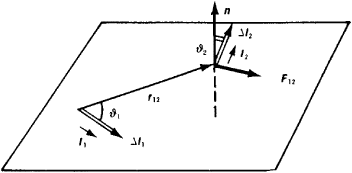Figure 1

The force F21 with which the second element acts on the first is expressed by a formula similar to equation (1). In absolute value the forces F12 and F21 are equal. However, in the general case where the orientation of Δl1 and Δ/2 with respect to one another is arbitrary, the directions of the forces F12 and F21 do not lie on the same straight line and do not comply with the principle of equal action and reaction.

In the special case of parallel conductors (see Figure 2) the forces of interaction tend to bring the conductors closer together if the currents flowing in them are parallel and to separate them if the currents are antiparallel. Thus, parallel currents attract, and antiparallel currents repel.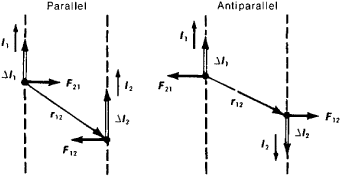Figure 2. Interaction of two elementary currents. All segments (vectors) in the figure lie in one plane

Ampére’s law is also known as the formula for determining the force F with which a magnetic field described by a magnetic induction vector B acts on an elementary segment of a conductor Δ/ in which a current of strength / is flowing:where ϑ is the angle between the directions of Δ/ and B. In the Gaussian system k = 1/c and in the SI system k = 1. Equation (2) is derived from equation (1) by extracting the part that does not contain the quantities relating to the second current element and by taking B to mean the magnetic induction created by the first current element at the point where the second current element is located (compare the Biot-Savart law).

With DC it is impossible to isolate a specific current element because a DC circuit is always a closed loop. The force of one current loop acting on another current loop or the force experienced by one current in a magnetic field created by another current can only be measured experimentally. This force is equal to the vector sum of the forces acting on each current element by the magnetic field of the other current. (In this case the magnetic field is the field resulting from all the current elements.) For the resultant forces experienced by interacting current loops, the principle of equal action and reaction turns out to be valid.

The standard for the unit of current strength, the ampere, is based on Ampère’s law and determined by using a current balance.

G. IA. MIAKISHEV

Mentioned in ?
References in periodicals archive ?
The Deriving Ampere's Law task, which is the realistic model-eliciting activity used in this study, was developed to extend the mathematics connections associated with the Solenoid Invention Kit.
It is the switched current that happens first, with the EM field being a consequence of Ampere's Law. The EM field does not induce the current; the current generates the EM field.
Using Ampere's Law, the ampere turns across the interwinding region is the same as the ampere turns in either winding.
607 (repeated in many textbooks) of the dynamic current equation with the J + D term begins with "Ampere's law" (see Art.
Grover starts from the basic Biot-Savart Law, which comes from Ampere's Law and Gauss's Law, each, one of Maxwell's equations, and derives all his approximations.
The magnitude of the Poynting's vector on the earth's surface S(r, 0) = [E.sub.r](r, 0)H(r, 0), for r > a may be calculated by considering the simple formula of the magnetic field intensity given by the Ampere's law H(r, 0) = i/ (2[pi]r).
While filling us in on why spending your allowance on x- ray glasses is a poor investment, Kakalios covers mechanics (including Newton's Law of Gravity, the properties of matter and the cube-square law) energy (including conservation and Ampere's Law), and modern physics (including quantum mechanics, solid-state physics and tunneling phenomena).
Ampere's Law (1825): An electric current is accompanied by a magnetic field whose direction is at right angles to the current flow.
So it is possible to rewrite Ampere's Law in function of vector "D".
[DELTA] x E = -j[omega]B Faraday's law [DELTA] x H = J + j[omega]D Ampere's law [DELTA] x D = [[rho].sub.lambda] Gauss' law [DELTA] x B = 0 Gauss' law (magnetic)
The currents flowing on the pair of half loops generate fluxes with opposite directions by the Ampere's law. By setting this loop coupler at the desired position, the [TM.sub.111] mode can be suppressed and [TE.sub.011] mode excited in one-port cylindrical cavity.
In FDTD, the electric field is stored at grid locations and the integration shown on the left of Ampere's law in Eq.

Site: Follow: Share:
Open / Close